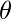# Exercise: Questions on Graph

Questions on Graph : Question 1 :
Which of the following is an advantage of adjacency list representation over adjacency matrix representation of a graph?

 In adjacency list representation, space is saved for sparse graphs. DFS and BSF can be done in O(V + E) time for adjacency list representation. These operations take O(V^2) time in adjacency matrix representation. Here is V and E are number of vertices and edges respectively. Adding a vertex in adjacency list representation is easier than adjacency matrix representation. All of the above  Correct
Questions on Graph : Question 2 :
The degree sequence of a simple graph is the sequence of the degrees of the nodes in the graph in decreasing order. Which of the following sequences can not be the degree sequence of any graph? I. 7, 6, 5, 4, 4, 3, 2, 1 II. 6, 6, 6, 6, 3, 3, 2, 2 III. 7, 6, 6, 4, 4, 3, 2, 2 IV. 8, 7, 7, 6, 4, 2, 1, 1

 I and II III and IV IV only II and IV  Correct
Questions on Graph : Question 3 :
The time complexity of computing the transitive closure of a binary relation on a set of n elements is known to be:

 O(n) O(nLogn) O(n ^ (3/2)) O(n^3)  Correct
Questions on Graph : Question 4 :
The most efficient algorithm for finding the number of connected components in an undirected graph on n vertices and m edges has time complexity. (A)(n) (B)(m) (C)(m + n) (D)(mn)

 A B C  Correct D
Questions on Graph : Question 5 :
Consider an undirected unweighted graph G. Let a breadth-first traversal of G be done starting from a node r. Let d(r, u) and d(r, v) be the lengths of the shortest paths from r to u and v respectively, in G. lf u is visited before v during the breadth-first traversal, which of the following statements is correct? (GATE CS 2001)

 d(r, u) < d (r, v) d(r, u) > d(r, v) d(r, u) <= d (r, v)  Correct None of the above
Questions on Graph : Question 6 :

 n(n-l)/2 2^n n! 2^(n(n-1)/2)  Correct
Questions on Graph : Question 7 :
Which of the following statements is/are TRUE for an undirected graph? P: Number of odd degree vertices is even Q: Sum of degrees of all vertices is even

 P Only Q Only Both P and Q  Correct Neither P nor Q
Questions on Graph : Question 8 :
Consider an undirected random graph of eight vertices. The probability that there is an edge between a pair of vertices is 1/2. What is the expected number of unordered cycles of length three?

 1/8 1 7  Correct 8